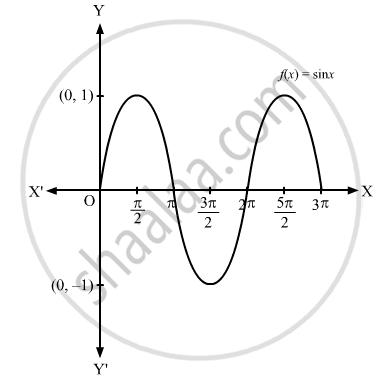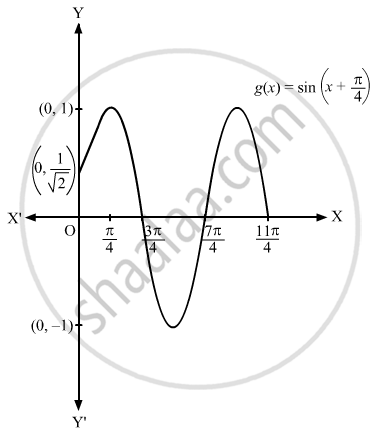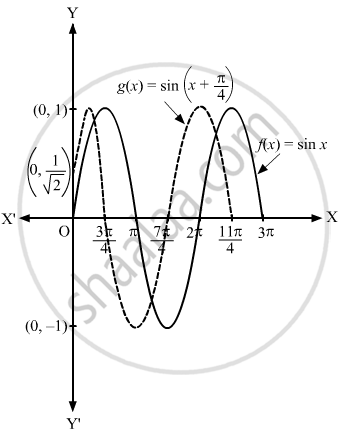Advertisement Remove all ads

# Sketch the Graph of the Following Pair of Functions on the Same Axes: F ( X ) = Sin X , G ( X ) = Sin ( X + π 4 ) - Mathematics

Answer in Brief

Sketch the graph of the following pair of functions on the same axes:
$f\left( x \right) = \sin x, g\left( x \right) = \sin \left( x + \frac{\pi}{4} \right)$

Advertisement Remove all ads

#### Solution

$f\left( x \right) = \sin\frac{x}{2}, g\left( x \right) = \sin x$
Clearly, sin x and $\sin \left( x + \frac{\pi}{4} \right)$ is a periodic function with period 2π.
The graphs of $f\left( x \right) = \sin x\text{ and }g\left( x \right) = \sin \left( x + \frac{\pi}{4} \right)$ on different axes are shown below:If these two graphs are drawn on the same axes, then the graph is shown below.Concept: Graphs of Trigonometric Functions
Is there an error in this question or solution?
Advertisement Remove all ads

#### APPEARS IN

RD Sharma Class 11 Mathematics Textbook
Chapter 6 Graphs of Trigonometric Functions
Exercise 6.1 | Q 2.1 | Page 5
Advertisement Remove all ads
Advertisement Remove all ads
Share
Notifications

View all notifications

Forgot password?